# Optimum Resistors Find Tools

This page is a web application that design circuits of resistors. Use this utility to find the optimum set of resistors for a circuit of resistors from the resistor sequence.

## Voltage Divider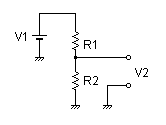V1 (Input Voltage) :V V2 (Desired Output Voltage) :V
Select Resistor Sequence:

## Voltage Divider2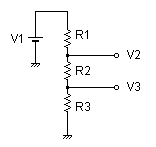V1 (Input Voltage) :V V2 (Desired Output Voltage) :V V3 (Desired Output Voltage) :V
Select Resistor Sequence:

## Parallel and Series of two Resistors

 Equivalent Resistor Ra Parallel Series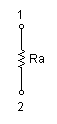→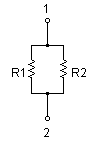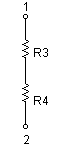Select Resistor Sequence:
Ra (Desired Resistance):
Ω (10-1000MΩ)

The Ra is resistance to place in parallel and in series of two resistors to create a non standard resistance. This tool find the optimum set of resistors R1,R2 and R3,R4 for a desired Ra value.

## Calcurate for resistances to divide a voltage (to branch a current)

 Equivalent Resistor Ra Parallel Series→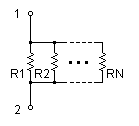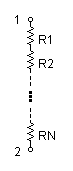Select Resistor Sequence:
 N (The total number of resistors) : (2-99) Ra (Desired Resistance) :Ω (10-1000MΩ)

Three More resistors circuit is often combined to divide a voltage (to branch a current). This tool find the optimum set of R1,R2…RN resistors for a desired Ra value from a close resistor value as even as combining resistances.